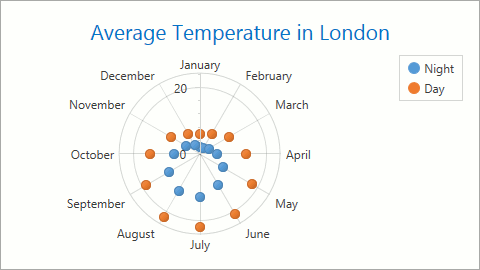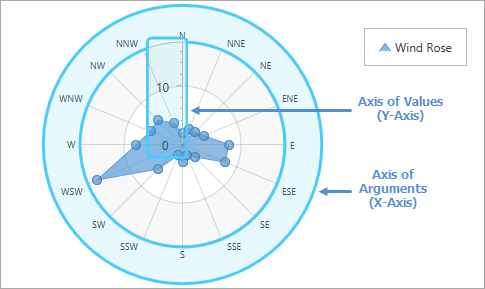20.1
19.2
19.1
18.2
18.1
17.2
The page you are viewing does not exist in version 17.2. This link will take you to the root page.

The 2D Radar Diagram is used to display data as a circular graph. A radar chart consists of a sequence of spokes dividing the diagram into equiangular sectors, where an each spoke represents one of the variables.The document includes the following sections:

## Compatible Series Types

This diagram can only contain the following compatible series types:

## How to Create a Chart with a Radar Diagram

The axis of arguments in the Radar diagram is represented as a circular axis. This axis is available with the RadarDiagram2D.AxisX property.##### NOTE

Note that radar diagram axes do not support additional elements such as constant lines, axis titles, and strips.

The following markup demonstrates how to create a chart with the Radar diagram:

``````<dxc:ChartControl>
ShapeStyle="Polygon"
DomainBrush="AliceBlue"
DomainBorderBrush="AliceBlue"
StartAngle="180"
LabelsResolveOverlappingMinIndent="5">
<!-- X-axis settings. -->
<!-- Y-axis settings. -->
<!-- The Radar series settings. -->
<dxc:SeriesPoint Argument="1/1/2017" Value="2"/>
<dxc:SeriesPoint Argument="2/1/2017" Value="2"/>
<dxc:SeriesPoint Argument="3/1/2017" Value="3"/>
<dxc:SeriesPoint Argument="4/1/2017" Value="5"/>
<dxc:SeriesPoint Argument="5/1/2017" Value="8"/>
<dxc:SeriesPoint Argument="6/1/2017" Value="11"/>
<dxc:SeriesPoint Argument="7/1/2017" Value="13"/>
<dxc:SeriesPoint Argument="8/1/2017" Value="13"/>
<dxc:SeriesPoint Argument="9/1/2017" Value="11"/>
<dxc:SeriesPoint Argument="10/1/2017" Value="8"/>
<dxc:SeriesPoint Argument="11/1/2017" Value="5"/>
<dxc:SeriesPoint Argument="12/1/2017" Value="3"/>
</dxc:ChartControl>
``````

The following table lists classes and properties that are used in the markup:

Class or Property Description
ChartControl The Chart control.
CircularDiagram2D.RotationDirection Specifies the direction in which the diagram's argument axis is drawn.
CircularDiagram2D.ShapeStyle Specifies the diagram's shape style.
CircularDiagram2D.DomainBrush Specifies the brush used to draw the diagram's area.
CircularDiagram2D.DomainBorderBrush Specifies the brush used to draw the diagram's area border.
CircularDiagram2D.StartAngle Specifies an angle of deflection from vertical to define an axis origin of coordinates.
CircularDiagram2D.LabelsResolveOverlappingMinIndent Specifies the minimum gap between two adjacent series point labels.
RadarDiagram2D.AxisX Gets or sets the x-axis settings.
RadarDiagram2D.AxisY Gets or sets the y-axis settings.
Series.DisplayName Specifies a series display name.
SeriesPoint The series point.
SeriesPoint.Argument Specifies the series point's argument.
SeriesPoint.Value Specifies the series point's value.

Refer to the code below to access the Radar diagram's settings at runtime:

``````// Cast Diagram to the RadarDiagram2D type.